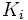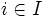# Compactly generated space

This article defines a property of topological spaces: a property that can be evaluated to true/false for any topological space|View a complete list of properties of topological spaces
This is a variation of compactness. View other variations of compactness

## Definition

### Symbol-free definition

A topological space is said to be compactly generated if the topology on it is generated by a collection of compact subsets. In other words, a set in the topological space is open if and only if its intersection with each of the compact subsets is open, in the subspace topology.

### Definition with symbols

A topological space$X$ is said to be compactly generated if there exists a collection$\{ K_i \}_{i \in I}$ of compact subsets of$X$, such that a subset$U \subset X$ is open if and only if$U \cap K_i$ is open in$K_i$ for every$i \in I$.

## Relation with other properties

### Stronger properties

Property Meaning Proof of implication Proof of strictness (reverse implication failure) Intermediate notions
compact space every open cover has a finite subcover compact implies compactly generated compactly generated not implies compact |FULL LIST, MORE INFO
locally compact space every point is contained in an open subset contained in a closed, compact subset locally compact implies compactly generated compactly generated not implies locally compact |FULL LIST, MORE INFO
first-countable space countable basis at every point first-countable implies compactly generated compactly generated not implies first-countable |FULL LIST, MORE INFO
metrizable space underlying topology of a metric space metrizable implies compactly generated compactly generated not implies metrizable |FULL LIST, MORE INFO
CW-space underlying topology of a CW-complex CW implies compactly generated compactly generated not implies CW |FULL LIST, MORE INFO
compactly generated Hausdorff space compactly generated and Hausdorff |FULL LIST, MORE INFO